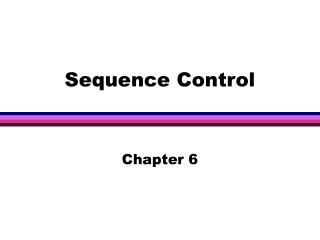DownloadDownload PresentationSequence Control

# Sequence Control

Download Presentation## Sequence Control

- - - - - - - - - - - - - - - - - - - - - - - - - - - E N D - - - - - - - - - - - - - - - - - - - - - - - - - - -
##### Presentation Transcript

1. Sequence Control Chapter 6

2. Control structures: the basic framework within which operations and data are combined into programs. • Sequence control • data control

3. Sequence control: the control of the order of execution of the operations (Primitive, user defined). • Data control: the control of the transmission of data among the subprograms of a program.

4. sequence control • Structures used in expressions (and thus within statements) such as precedence rules , parentheses. • Structures used between statements or groups of statements, suchas conditional , iteration. • Structures used between subprograms, such as subprograms calls , coroutines.

5. Implicit sequence control: (default) defined by the language . • Explicit sequence control: defined by the programmer.

6. 6.2. Sequence with arithmetic expressions • R=(-B+SQRT(B**2-4*A*C))/(2*A) • 15 separate operations • can the two references to the value of B and A be combined? • what order to evaluate the expression to minimize the temporary storage ?

7. Tree-structure representation (p.240, fig. 6.1.) • syntax for expression • prefix notation no parentheses • postfix notation no parentheses • infix notation • semantics for expressions • evaluation of expressions (p.243)

8. Semantics for expressions • Hierarchy of operations (precedence rules) p.245 • Associativity (left to right ,right to left) p.245,246

9. Execution-Time Representation • Machine code sequences. • tree structures. • prefix or postfix form.

10. Evaluation of tree representation of expressions • Problems: • uniform evaluation rules • Side effects • error conditions • Short-circuit boolean expressions

11. uniform evaluation rules • Eager evaluation rule: first evaluate the operands (order is not important). P.250 • lazy evaluation rule: never evaluate the operands first. Fig. 6.4. Z+(X==o?Y:Y/X)

12. Z+(X=0?Y:Y/X) • pass the operands (or at least the last two operands) to the conditional operation unevaluated an let the operation determine the order of evaluation. • Passing parameters by value or by name

13. No simple evaluation rule • In LISP: functions are split into two categories, • receives evaluated operands • receives unevaluated operands • In SNOBOL4: • programmer-defined operations: receives evaluated operands, • language-defined operations: receives unevaluated operands.

14. Side effects • a*fun(x)+a • evaluate each term in sequence • evaluate a only once • call fun(x) before evaluating a

15. Side effects • solutions: • side effects should be outlawed in expressions, • language definition clears the order of evaluation, cause optimization impossible, • ignore the question, decided by implementer .

16. error conditions • Overflow, divide by zero. • Solutions varies from language to language and implementation to implementation.

17. Short-circuit Boolean expressions If ((A==0)||(B/A>c)) while ((I<=UB)&&(V[I]>c)) • in many languages both operands are evaluated before the Boolean operation is evaluated. • Solution in Ada, explicitly: • and then , or else

18. 6.3. Sequence with non-arithmetic expressions • Pattern matching • term rewriting • unification • backtracking

19. Pattern matching • Pattern matching by string replacement (in SNOBOL4 ): A-> 0A0 | 1A1 | 0 | 1 00100 A1 matches the center 1 A2 matches 0A10 A3 matches 0A20

20. In Prolog, a relation as a set of n-tuples, • specify known instances of these relations (called facts), • other instances can be derived.

21. ParentOf(John,Mary). ParentOf(Susan,Mary). ParentOf(Bill,John). ParentOf(Ann,John). ParentOf(X,Mary) ParentOf(X,Mary), ParentOf(Y,Mary), not(X=Y)

22. Building relations out of other relations, GrandparentOf(X,Y):- ParentOf(X,Z), ParentOf(Z,Y).

23. Term Rewriting • A restricted form of pattern matching • string: a1a2 …an • rewrite rule x=>y • if x=ai ,a1…ai-1y…an is a term rewrite of a1a2 …an.

24. Unification • Prolog uses unification, or the substitution of variables in relations, to pattern match. • Determine if the query has a valid substitution consistent with the rules and facts in the database.

25. A rule: • GrandparentOf(X,Y) :- ParentOf(X,Z), ParentOf(Z,Y) • ParentOf(X,Mary) = ParentOf(John,Y) • ParentOf(John,Mary) unifies it.

26. In Prolog : • Queries are unified with rules or with facts in the database until true results. • If false results, it means that a wrong rule or fact is used, then an alternative (if any) must be tried.

27. 6.4. Sequence control between statements • Basic statements • assignments (p. 265) • subprogram calls • I/O statements

28. Forms of statement-level sequence control • Composition • Alternation • Iteration

29. Explicit sequence control • goto • conditional • unconditional • break , continue (in C)

30. Structured programming design • Gotos advantages: • hardware support • easy to use in small programs • familiar to older programmers(!!) • general purpose

31. Gotos disadvantages: • lack of hierarchical program structure • there is no one-in, one-out control structure • spaghetti code (program text, execution order) • groups of statements serve multiple purposes

32. Structured Programming • hierarchical program design using only THE three structures. • Hierarchical Implementation like design. • No spaghetti code, textual sequence of statements like execution sequence. • groups of statements serve single purpose.

33. Structured sequence control • Compound statements • conditional statements • if (single-branch,multi-branch), case (p.274) . • Iteration statements

34. Iteration statements Head and a body (p.276,277) • Simple repetition • repetition while condition holds • repetition while incrementing counter • infinite repetition When is the termination test made? When are the variables used in the statement ahead evaluated?

35. In C • simple counter from 1 to 1o • for(I=1;I<=10;I++){body} • infinite loop • for(;;){body} • counter with exit condition • for(I=1;I<=100&&NotEntfile;I++){body}

36. Problems in structured sequence control • Multiple exit loops. • do-while-do. • exceptional conditions. p. 278,279